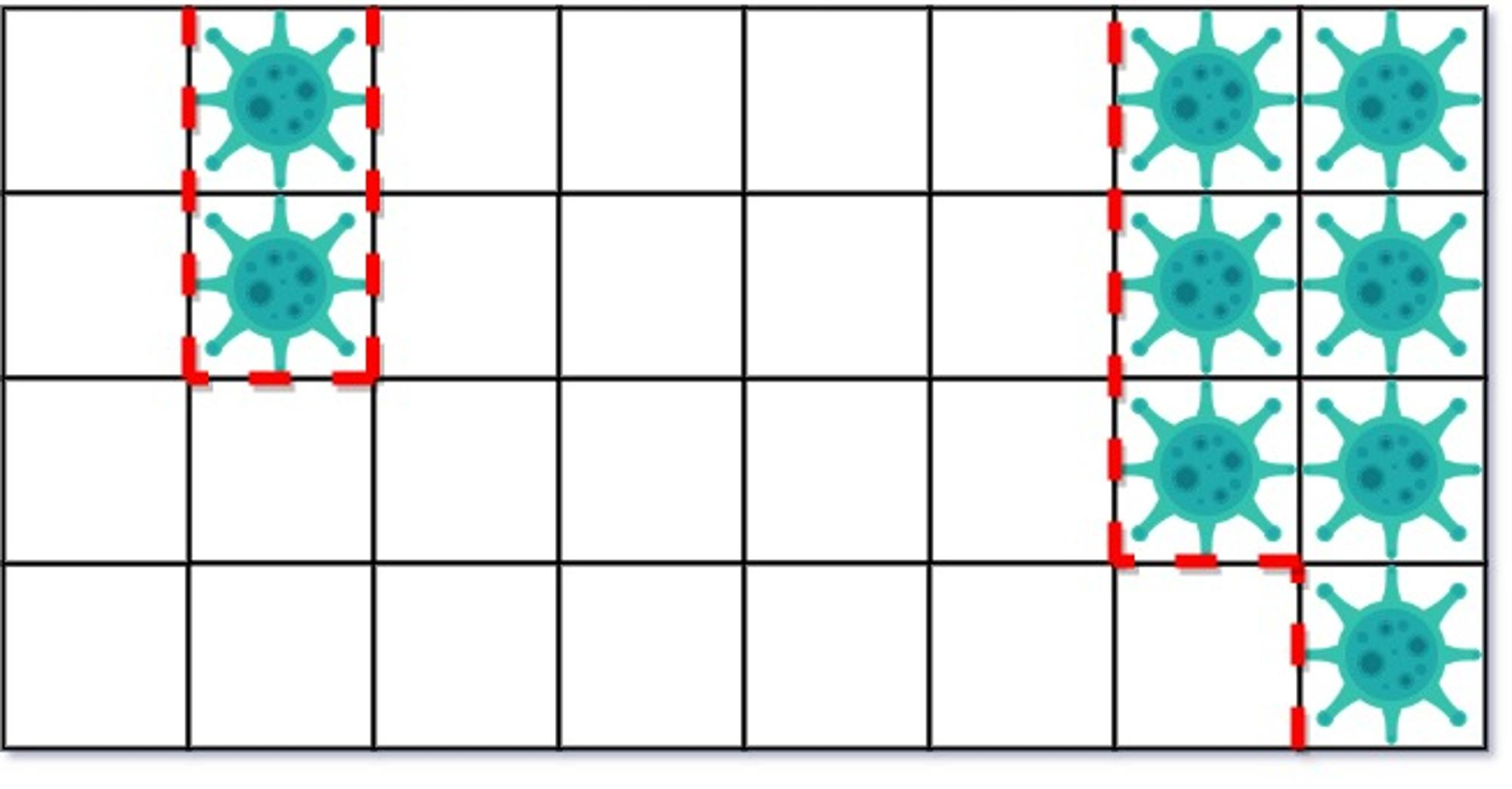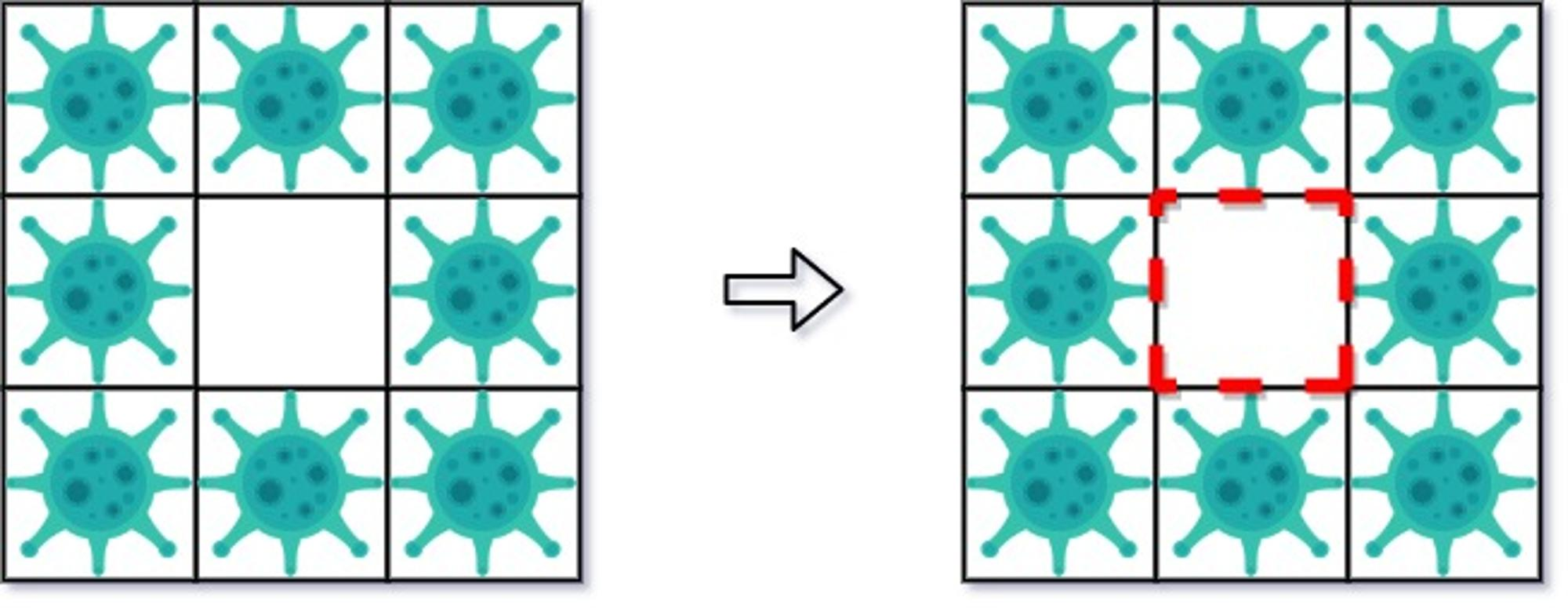V1

2022/07/18阅读：20主题：前端之巅同款

# 749. 隔离病毒 : 搜索模拟题

## 题目描述

Tag : 「模拟」、「图论搜索」、「BFS」

输入: isInfected = [[0,1,0,0,0,0,0,1],[0,1,0,0,0,0,0,1],[0,0,0,0,0,0,0,1],[0,0,0,0,0,0,0,0]]输出: 10解释:一共有两块被病毒感染的区域。在第一天，添加 5 墙隔离病毒区域的左侧。病毒传播后的状态是:第二天，在右侧添加 5 个墙来隔离病毒区域。此时病毒已经被完全控制住了。示例 2：输入: isInfected = [[1,1,1],[1,0,1],[1,1,1]]输出: 4解释: 虽然只保存了一个小区域，但却有四面墙。注意，防火墙只建立在两个不同区域的共享边界上。

输入: isInfected = [[1,1,1,0,0,0,0,0,0],[1,0,1,0,1,1,1,1,1],[1,1,1,0,0,0,0,0,0]]输出: 13解释: 在隔离右边感染区域后，隔离左边病毒区域只需要 2 个防火墙。

• isInfected[i][j] is either 0 or 1
• 在整个描述的过程中，总有一个相邻的病毒区域，它将在下一轮 严格地感染更多未受污染的方块

## 搜索模拟

• 找出当天「对未感染区域的威胁最大」的区域，并将该区域进行隔离（将 设置为 ）；
• 对其他区域，进行步长为 的感染操作。

BFS 过程中，我们除了统计该连通块所需要的防火墙数量 以外，还需要额外记录当前连通块中 的点集 s1（简称为原集，含义为连通块的格子集合），以及当前连通块相邻的 的点集 s2（简称为扩充集，含义为将要被感染的格子集合）。

Java 代码：

class Solution {    int[][] g;    int n, m, ans;    int[][] dirs = new int[][]{{1,0},{-1,0},{0,1},{0,-1}};    boolean[][] vis;    int search(int _x, int _y, Set<Integer> s1, Set<Integer> s2) {        int ans = 0;        Deque<int[]> d = new ArrayDeque<>();        vis[_x][_y] = true;        d.addLast(new int[]{_x, _y});        s1.add(_x * m + _y);        while (!d.isEmpty()) {            int[] info = d.pollFirst();            int x = info, y = info;            for (int[] di : dirs) {                int nx = x + di, ny = y + di, loc = nx * m + ny;                if (nx < 0 || nx >= n || ny < 0 || ny >= m || vis[nx][ny]) continue;                if (g[nx][ny] == 1) {                    s1.add(loc);                    vis[nx][ny] = true;                    d.addLast(new int[]{nx, ny});                } else if (g[nx][ny] == 0) {                    s2.add(loc);                    ans++;                }            }        }        return ans;    }    int getCnt() {        vis = new boolean[n][m];        int max = 0, ans = 0;        // l1: 每个连通块的点集 s2: 每个连通块的候选感染点集        List<Set<Integer>> l1 = new ArrayList<>(), l2 = new ArrayList<>();        for (int i = 0; i < n; i++) {            for (int j = 0; j < m; j++) {                if (g[i][j] == 1 && !vis[i][j]) {                    // s1: 当前连通块的点集 s2: 当前连通块的候选感染点集                    Set<Integer> s1 = new HashSet<>(), s2 = new HashSet<>();                    int b = search(i, j, s1, s2), a = s2.size();                    if (a > max) {                        max = a; ans = b;                    }                    l1.add(s1); l2.add(s2);                }            }        }        for (int i = 0; i < l2.size(); i++) {            for (int loc : l2.get(i).size() == max ? l1.get(i) : l2.get(i)) {                int x = loc / m, y = loc % m;                g[x][y] = l2.get(i).size() == max ? -1 : 1;            }        }        return ans;    }    public int containVirus(int[][] _g) {        g = _g;        n = g.length; m = g.length;        while (true) {            int cnt = getCnt();            if (cnt == 0) break;            ans += cnt;        }        return ans;    }}

TypeScript 代码：

let g: number[][] = nulllet n: number = 0, m: number = 0let vis: boolean[][] = nullconst dirs: number[][] = [[1,0],[-1,0],[0,1],[0,-1]]function dfs(_x: number, _y: number, s1: Set<number>, s2: Set<number>): number {    let he = 0, ta = 0, ans = 0    let d: Array<number> = new Array<number>()    s1.add(_x * m + _y)    vis[_x][_y] = true    d[ta++] = _x * m + _y    while (he < ta) {        const poll = d[he++]        const x = Math.floor(poll / m), y = poll % m        for (const di of dirs) {            const nx = x + di, ny = y + di, loc = nx * m + ny            if (nx < 0 || nx >= n || ny < 0 || ny >= m || vis[nx][ny]) continue            if (g[nx][ny] == 1) {                s1.add(loc)                vis[nx][ny] = true                d[ta++] = loc            } else if (g[nx][ny] == 0) {                s2.add(loc)                ans++            }        }    }    return ans}function getCnt(): number {    vis = new Array<Array<boolean>>(n)    for (let i = 0; i < n; i++) vis[i] = new Array<boolean>(m).fill(false)    let max = 0, ans = 0    let l1: Array<Set<number>> = new Array<Set<number>>(), l2: Array<Set<number>> = new Array<Set<number>>()    for (let i = 0; i < n; i++) {        for (let j = 0; j < m; j++) {            if (g[i][j] == 1 && !vis[i][j]) {                let s1 = new Set<number>(), s2 = new Set<number>()                const b = dfs(i, j, s1, s2), a = s2.size                if (a > max) {                    max = a; ans = b                }                l1.push(s1); l2.push(s2)            }        }    }    for (let i = 0; i < l2.length; i++) {        for (let loc of l2[i].size == max ? l1[i] : l2[i]) {            const x = Math.floor(loc / m), y = loc % m            g[x][y] = l2[i].size == max ? -1 : 1        }    }    return ans}function containVirus(_g: number[][]): number {    g = _g    n = g.length; m = g.length    let ans: number = 0    while (true) {        const cnt = getCnt()        if (cnt == 0) break        ans += cnt    }    return ans};
• 时间复杂度：最多有 天需要模拟，每天模拟复杂度 ，整体复杂度为
• 空间复杂度：

## 最后V1